GATE  >  Test: Soil Mechanics

# Test: Soil Mechanics

Test Description

## 25 Questions MCQ Test GATE Civil Engineering (CE) 2023 Mock Test Series | Test: Soil Mechanics

Test: Soil Mechanics for GATE 2023 is part of GATE Civil Engineering (CE) 2023 Mock Test Series preparation. The Test: Soil Mechanics questions and answers have been prepared according to the GATE exam syllabus.The Test: Soil Mechanics MCQs are made for GATE 2023 Exam. Find important definitions, questions, notes, meanings, examples, exercises, MCQs and online tests for Test: Soil Mechanics below.
Solutions of Test: Soil Mechanics questions in English are available as part of our GATE Civil Engineering (CE) 2023 Mock Test Series for GATE & Test: Soil Mechanics solutions in Hindi for GATE Civil Engineering (CE) 2023 Mock Test Series course. Download more important topics, notes, lectures and mock test series for GATE Exam by signing up for free. Attempt Test: Soil Mechanics | 25 questions in 75 minutes | Mock test for GATE preparation | Free important questions MCQ to study GATE Civil Engineering (CE) 2023 Mock Test Series for GATE Exam | Download free PDF with solutions
 1 Crore+ students have signed up on EduRev. Have you?
Test: Soil Mechanics - Question 1

### The shear strength parameters of the soil are C = 15kN/m2 and φ = 200. Undrained triaxial test is carried on the specimen of the soil. Determine the deviator stress at which failure will occur if the cell pressure is 200 kN/m2. The pore pressure developed during the test is 50 kN/m2.

Detailed Solution for Test: Soil Mechanics - Question 1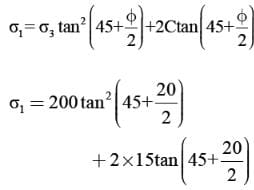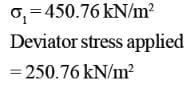*Answer can only contain numeric values
Test: Soil Mechanics - Question 2

### Consider a square footing 2m × 2m located at a depth of 1m below the ground level. The load dispersion below the base of footing is 2V : 1H.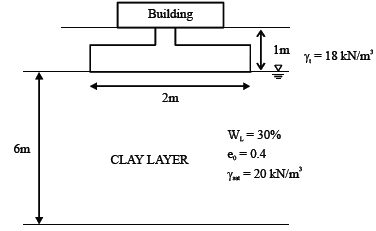The pressure acting at the base of footing due to erection of building is 100 KPa, find the  consolidation settlement of foundation (in mm).________ (Important - Enter only the numerical value in the answer)

Detailed Solution for Test: Soil Mechanics - Question 2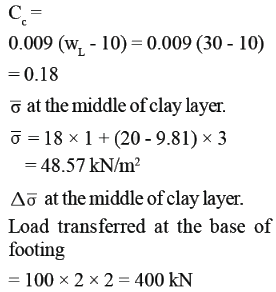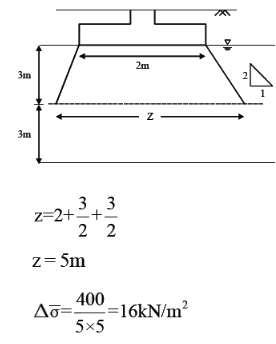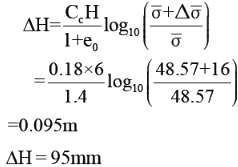*Answer can only contain numeric values
Test: Soil Mechanics - Question 3

### For the given soil profile, calculate the active earth pressure at point A.____kN/m2 Unit weight of soil is 10 kN/m3.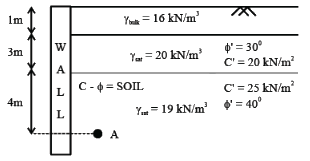(Important - Enter only the numerical value in the answer)

Detailed Solution for Test: Soil Mechanics - Question 3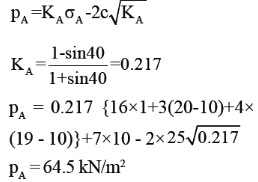Test: Soil Mechanics - Question 4

Difference between upstream and downstream water levels for a weir is 3m. The discharge per metre width of sheet pile is K = 0.2 m2/s. Coefficient of permeability for soil at base of weir is 0.1 m/s. Calculate the number of flow lines when the number of equipotential head drops is 12?

Detailed Solution for Test: Soil Mechanics - Question 4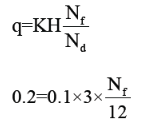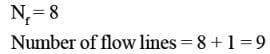Test: Soil Mechanics - Question 5

In an aquifer extending over 200 Hectares, the water table was 20m below the ground level. The specific yield and specific retention of aquiter are 0.15 and 0.25 respectively. If due to rainfall the water table rises by 2m, calculate the change in storage of aquifer?

Detailed Solution for Test: Soil Mechanics - Question 5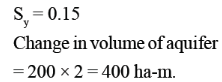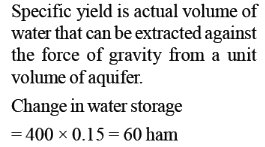*Answer can only contain numeric values
Test: Soil Mechanics - Question 6

A 20m thick clay layer is aandwiched between gravelly layer at the top and impermeable layer at the bottom. The layer experiences 10mm settlement in 2 years. If coefficient of consolidation is 0.004 cm2/s, the deposit will experience a total of 15mm settlement in next ____ years.

(Important - Enter only the numerical value in the answer)

Detailed Solution for Test: Soil Mechanics - Question 6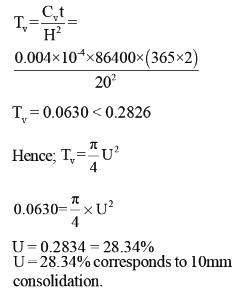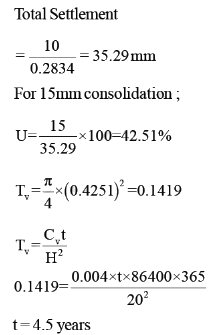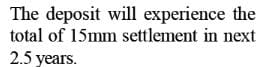*Answer can only contain numeric values
Test: Soil Mechanics - Question 7

In a consolidated drained triaxial test, a specimen of clay tails at a cell pressure of 100kN/m2. The effective shear strength parameters are c' = 20kN/m2 and φ' = 300.

Determine the major principal stress?___________kN/m2

(Important - Enter only the numerical value in the answer)

Detailed Solution for Test: Soil Mechanics - Question 7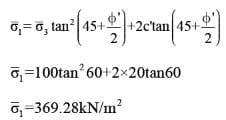Test: Soil Mechanics - Question 8

A soil sample has a mass of 3kg and volume of 0.0016 m3 in natural condition. After being oven dried in an oven, the mass of the sample is  2.6kg. Specific gravity (G) is 2.7. Unit weight of water is 10 kN/m3. The air content of the soil is:

Detailed Solution for Test: Soil Mechanics - Question 8

Water content (w)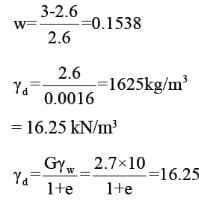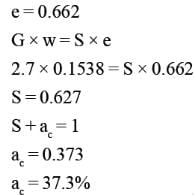Test: Soil Mechanics - Question 9

The void ratio at the densest, loosest and natural states of a sand deposit are 0.3, 0.8 and 0.4 respectively.
The relative density of the deposit is :

Detailed Solution for Test: Soil Mechanics - Question 9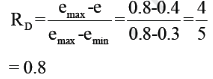Test: Soil Mechanics - Question 10

Sieve analysis on a soil sample showed 40% of the soil passed through 4.75mm sieve and 8% of the soil passed through 75μ sieve. The liquid limit and plastic limit of soil are 40% and 24% respectively. The soil may be classified as:

Detailed Solution for Test: Soil Mechanics - Question 10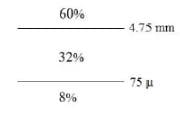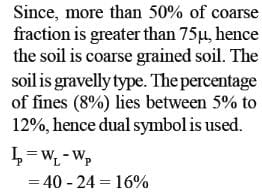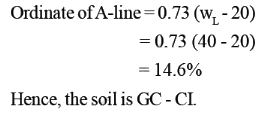*Answer can only contain numeric values
Test: Soil Mechanics - Question 11

Two identical soil samples are subjected to falling head permeability test in laboratory under identical  conditions. The fall of head was measured after t sec in 1st specimen and it was observed that ratio of initial to final water heads was 1.5. The fall of head was measured after 2t seconds in 2nd specimen and it was observed that ratio of initial to final water heads was 5. Find the ratio of coefficient of permeability of specimen 1 to specimen 2?

(Important - Enter only the numerical value in the answer)

Detailed Solution for Test: Soil Mechanics - Question 11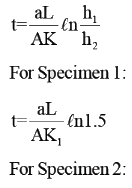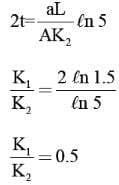*Answer can only contain numeric values
Test: Soil Mechanics - Question 12

Water flows from A to B through two soil samples. The rectangular cross section of the two soil layers have depth of 10cm and 20cm respectively and inside width of 15cm. Over a period of 1 hours, volume of water collected through section PQ is 3600 mL. The flow condition can be assumed to be steady state. If the coefficient of permeability of layer 1 is 2 × 10-5 m/s, the coefficient of permeability through layer 2 is ____kN.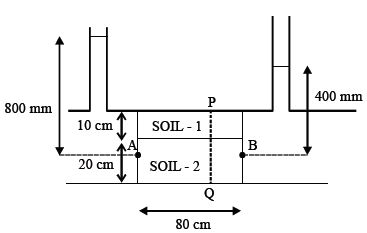(Important - Enter only the numerical value in the answer and express the amswer in decimal form only)

Detailed Solution for Test: Soil Mechanics - Question 12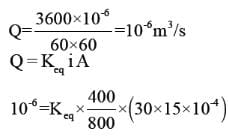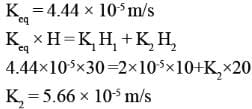Test: Soil Mechanics - Question 13

The sand layer is found at 10m depth below the sea water level. The void ratio and specific gravity of soil solid is 0.4 and 2.7. The specific gravity of sea water is 1.1 and unit weight of fresh water is 9.81 kN/m3. Find the effective stress at 20m depth into the sand layer?

Detailed Solution for Test: Soil Mechanics - Question 13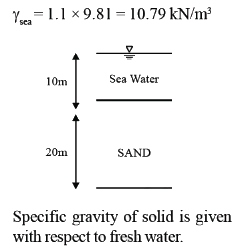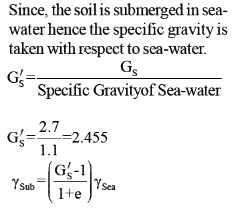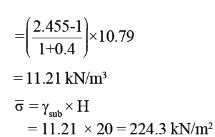Test: Soil Mechanics - Question 14

The specific gravity and in-situ void ratio of soil deposit are 2.67 and 0.8 respectively. The value of critical hydraulic gradient is:

Detailed Solution for Test: Soil Mechanics - Question 14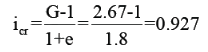Test: Soil Mechanics - Question 15

The virgin compression curve for a soil is shown in the figure below. What is the compression index of the soil?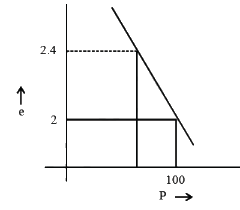Detailed Solution for Test: Soil Mechanics - Question 15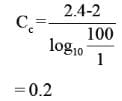Test: Soil Mechanics - Question 16

A vertical cut is to be made in a saturated clay with C = 15 kN/m2, φ = 300 and γt = 18 kN/m3. What is
the theoretical depth to which the clay can be excavated without side collapse?

Detailed Solution for Test: Soil Mechanics - Question 16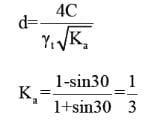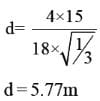*Answer can only contain numeric values
Test: Soil Mechanics - Question 17

A slope is to be constructed at an angle of 300 to the horizontal from a soil having properties, e = 20 kN/ m2, φ = 300, γ= 19 kN/m3. Taylor’s stability numbers is 0.05. If the factor of safety of 1.5 is required, then the safe height of the slope (in m) will be______

(Important - Enter only the numerical value in the answer)

Detailed Solution for Test: Soil Mechanics - Question 17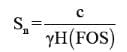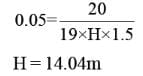*Answer can only contain numeric values
Test: Soil Mechanics - Question 18

A deposit of sand has a porosity of 35% and G = 2.7. The soil is dry in the top 2m depth, it has 20% moisture content in the next 2m depth and it is submerged below it. Find the effective stress at 5m below the ground surface.__________kN/m2.

(Important - Enter only the numerical value in the answer)

Detailed Solution for Test: Soil Mechanics - Question 18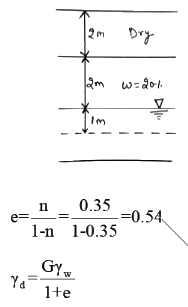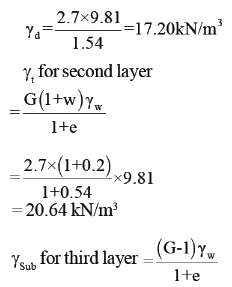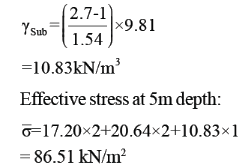Test: Soil Mechanics - Question 19

The soil is compacted at the dry side of optimum. The sensitivity of the soil compacted at dry side when
compared to compacted at wet side is?

*Answer can only contain numeric values
Test: Soil Mechanics - Question 20

In a 8m thick stratum of fine sand having submerged density of 11kN/m3, excavation is to be made. The water table is at a depth of 2m below the ground level. Calcuate the maximum permissible depth of excavation such that there is no quick sand condition possible? Take density of water as 10 kN/m3. During excavation, dewatering operation is also carried out: _____m.

(Important - Enter only the numerical value in the answer)

Detailed Solution for Test: Soil Mechanics - Question 20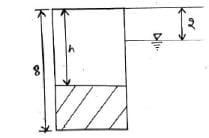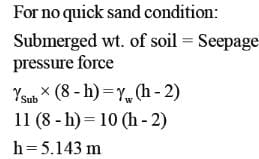Test: Soil Mechanics - Question 21

A soil fails under an axial vertical stress of 200kN/m2 in unconfined compression test. The failure plane makes an angle of 500 with horizontal. The shear parameter ‘c’ and ‘φ’ respectively will be:

Detailed Solution for Test: Soil Mechanics - Question 21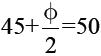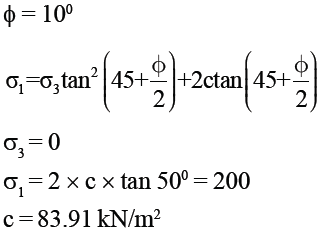Test: Soil Mechanics - Question 22

A clay sample is tested in triaxial apparatus in consolidated drained conditions at a cell pressure of 100 kN/m2. What will be the pore water pressure at a deviator stress of 60 kN/m2?

Detailed Solution for Test: Soil Mechanics - Question 22

In consolidated drained test pore water pressure is zero in both the stages.

*Answer can only contain numeric values
Test: Soil Mechanics - Question 23

A square footing (4m × 4m) is subjected to an inclined point load P, as shown in the figure below. The water table is located at a great depth below the base of footing. Considering two way eccentricity that is ex = 0.2m and ey = 0.3m, the net safe load carrying capacity of the footing for a factor of safety of 2.5 is ____kN.
The following factors may be used:

Bearing Capacity factor :

Nq = 34,

Nγ = 25

Shape factors : Fqs = Fγs = 1.5

Depth factors : Fqd = Fγd = 1.2

Inclination Factors : Fqi = 0.4, Fγi = 0.04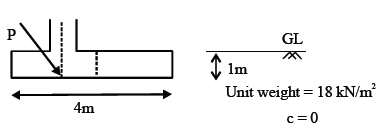(Important - Enter only the numerical value in the answer)

Detailed Solution for Test: Soil Mechanics - Question 23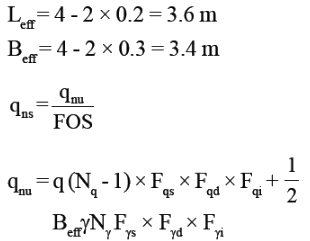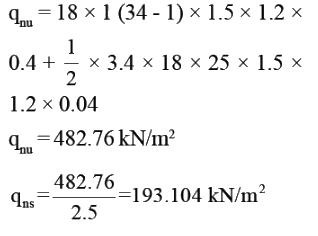For two way eccentricity, the
reduced area is taken as: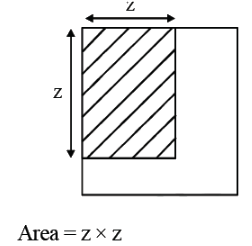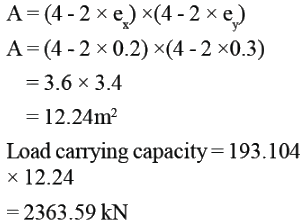*Answer can only contain numeric values
Test: Soil Mechanics - Question 24

The square footing (2m × 2m) is located at a depth of 1.5m from the ground level. Unit weight of soil γt = 20 kN/m3. The backfilling is not done after laying the footing.

Find the safe load that can be carried by footing considering the factor of safety of 3.

φ0= 400 → Nq = 10, Nγ= 6 ; c = 0  ___________________ kN

(Important - Enter only the numerical value in the answer)

Detailed Solution for Test: Soil Mechanics - Question 24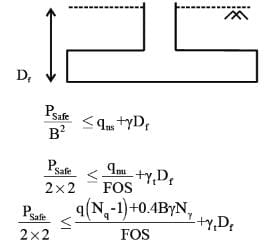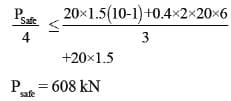Test: Soil Mechanics - Question 25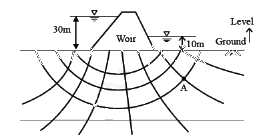Calculate the effective stress at point A 12m below the Ground level. The Saturated bulk unit weight of soil is 20 kN/m3 and unit weight of water is 10 kN/m3.

Detailed Solution for Test: Soil Mechanics - Question 25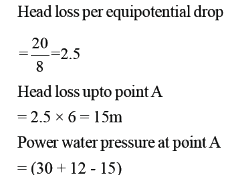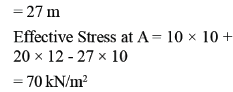## GATE Civil Engineering (CE) 2023 Mock Test Series

26 docs|292 tests
 Use Code STAYHOME200 and get INR 200 additional OFF Use Coupon Code
Information about Test: Soil Mechanics Page
In this test you can find the Exam questions for Test: Soil Mechanics solved & explained in the simplest way possible. Besides giving Questions and answers for Test: Soil Mechanics, EduRev gives you an ample number of Online tests for practice

## GATE Civil Engineering (CE) 2023 Mock Test Series

26 docs|292 tests